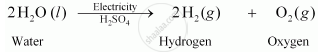# In Electrolysis of Water, Why is the Volume of Gas Collected Over One Electrode Double that of Gas Collected Over the Other Electrode? - Science

#### Question

In electrolysis of water, why is the volume of gas collected over one electrode double that of gas collected over the other electrode?

#### Solution

The balanced chemical equation for the electrolysis of water is:It can be observed from the balanced chemical equation that the volumes of hydrogen and oxygen produced during electrolysis are in the ratio 2: 1. Thus, the volume of hydrogen gas collected in the process is double than that of oxygen.

Is there an error in this question or solution?

#### Video TutorialsVIEW ALL 

In Electrolysis of Water, Why is the Volume of Gas Collected Over One Electrode Double that of Gas Collected Over the Other Electrode? Concept: Balanced Chemical Equation.